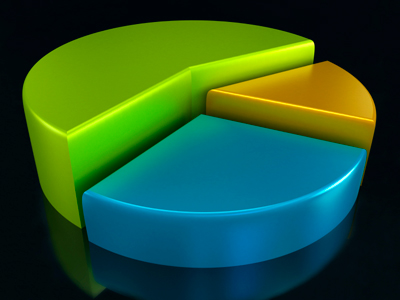Formulae can create output as charts and graphs like a pie chart.

# Models 02

This KS3 ICT quiz is our second on models. Spreadsheets are frequently used for financial information because of their ability to re-calculate the entire sheet automatically after a change to a single cell is made. This is a simple way for businesses to see how small changes to prices or sales could affect their profits and losses.

Models work by taking a set of data and using rules to see how things change. The rules are represented using formulae, for example, profit is equal to the total sales minus the total cost of the wages, raw materials and other expenses. The data required to calculate the result of this formula will be contained in other cells. These can be read into the formula by making reference to the addresses of the other cells. In our example above, if the total sales is in cell B1, the wages figure in cell F8 and the total of the raw materials and other expenses in cell G14, the formula would be =B1-(F8+G14). The parentheses tell the spreadsheet to add the wages and expenses before subtracting them from the total sales.

1.
Which of these is not a formula?
=C3+F2*H5
=C3-F2*\$H5
-C3/F2*H5
=C3*F2*H5
2.
Models represent real life situations using .......
data and analysis
data and intuition
data and rules
data and statistics
The rules decide what happens to the data
3.
Spreadsheet models use ....... to represent rules.
cells
conditional formatting
formulae
symbols
The formulae need to be set up very carefully to avoid incorrect results
4.
The values in the spreadsheet cells act as .......
information
input
macros
reports
These can be entered manually or calculated from data that has been entered into other cells
5.
The formulae in the models act as .......
calculations
data
enquiries
processes
These are effectively the rules that dictate how a model works. If just one of these is wrong, the whole model will fail
6.
The formulae can create output as charts and graphs.
Charts and graphs are the only form of output possible
Charts and graphs are vital to understand predictions
Charts and graphs can improve understanding of results
Charts and graphs make the output more accurate
Use them when they are useful - not just for looks
7.
Spreadsheets are good at doing ....... analysis of data.
how-why
what-else
what-if
why-when
For example: What happens to sales if tax rates increase?
8.
A model rule is: profit = number sold x saleprice - cost.
A suitable formula might be: =D5x(C5-B5)
A suitable formula might be: =D5x(C5/B5)
A suitable formula might be: =D5*(C5-B5)
A suitable formula might be: =D5^(C5-B5)
The 'rule' is represented by a formula entered in a cell
9.
Models often include many rules because .......
a single formula can only handle simple rules
real world situations are often very complicated
results can be achieved much quicker with more rules
the more rules used, the more accurate the results
Even simple situations can need many rules to model
10.
Predictions made by models are usually .......
approximate
correct
exact
wrong
Rules are rarely perfect and may not have complete data